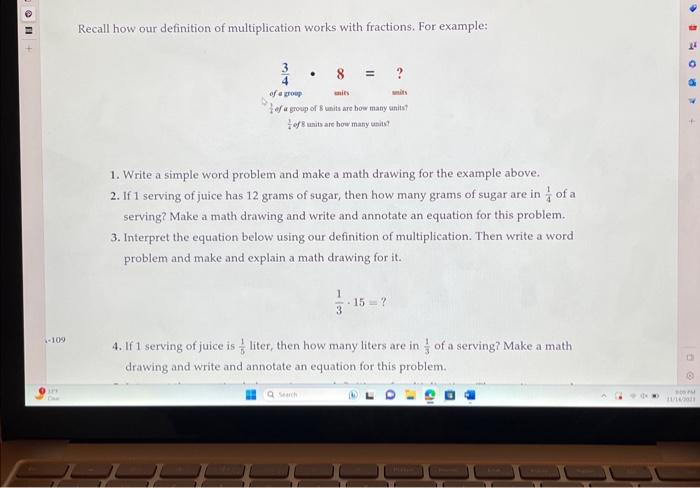Home / Expert Answers / Other Math / recall-how-our-definition-of-multiplication-works-with-fractions-for-example-1-write-a-simple-wo-pa766

# (Solved): Recall how our definition of multiplication works with fractions. For example: 1. Write a simple wo ...Recall how our definition of multiplication works with fractions. For example: 1. Write a simple word problem and make a math drawing for the example above. 2. If 1 serving of juice has 12 grams of sugar, then how many grams of sugar are in of a serving? Make a math drawing and write and annotate an equation for this problem. 3. Interpret the equation below using our definition of multiplication. Then write a word problem and make and explain a math drawing for it. 4. If 1 serving of juice is liter, then how many liters are in of a serving? Make a math drawing and write and annotate an equation for this problem.

We have an Answer from Expert